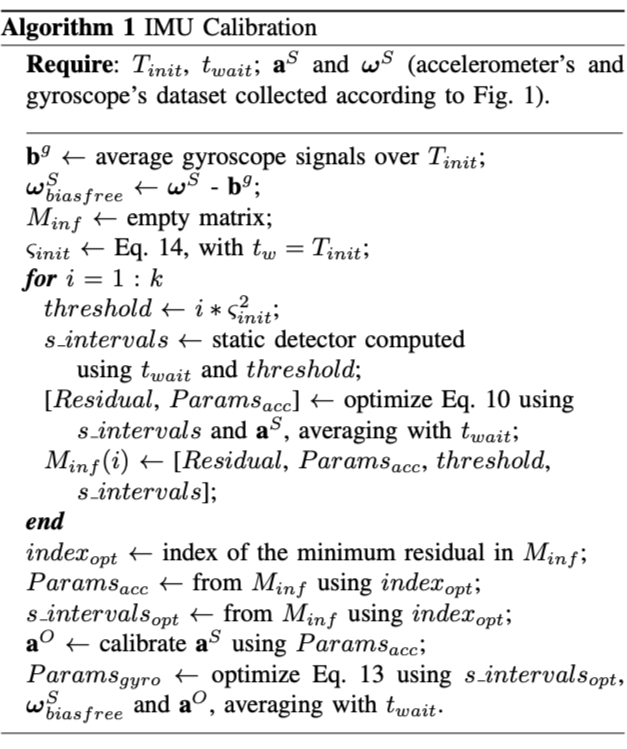# A Robust and Easy to Implement Method for IMU Calibration without External Equipments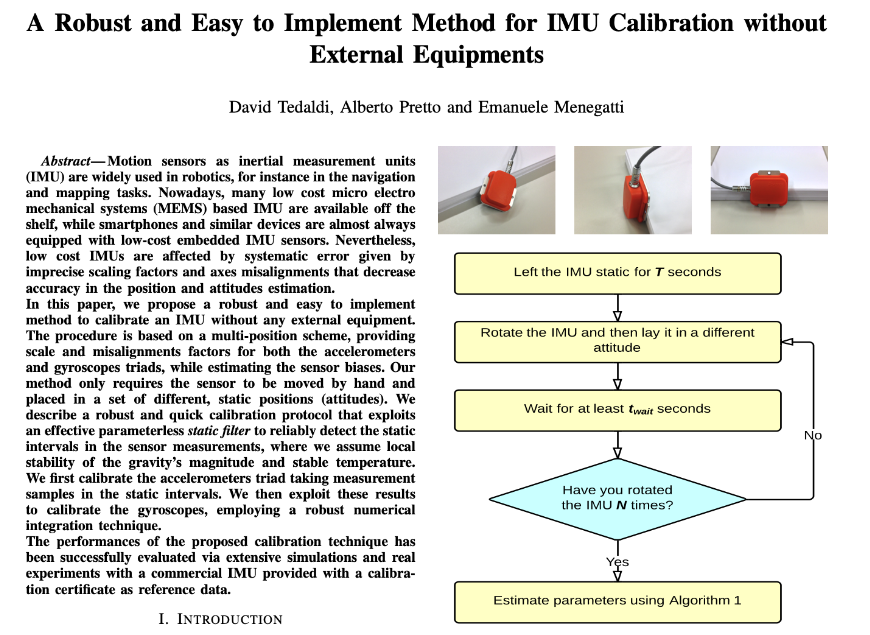# 介绍

IMU标定，其实就是对上述参数的辨识过程。

# 传感器误差模型

• AF：加速度计坐标系（非正交）
• GF：陀螺仪坐标系（非正交）
• AOF：与AF坐标系关联的正交坐标系
• 其中，AOF的x轴与AF的重合
• AOF的y轴处于AF坐标系中x轴与y轴所张成的平面中
• GOF：类似同理
• BF：载体坐标系（正交）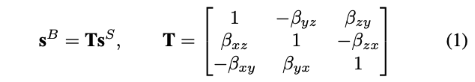• $$s^{\mathcal{B}},s^{\mathcal{S}}$$可以是加速度或者角速度，分别在载体坐标系和加速度计(陀螺仪)坐标系的表示
• $$\beta_{ij}$$表示加速度计的第i轴绕载体坐标系的第j轴的旋转，如下图2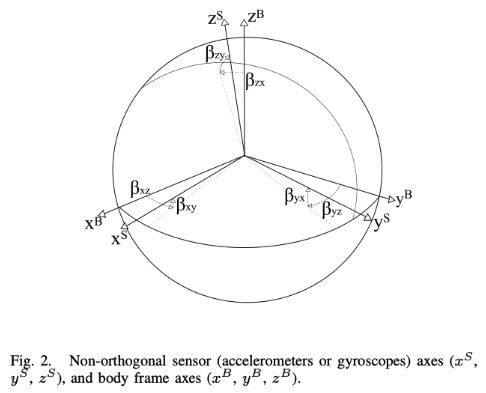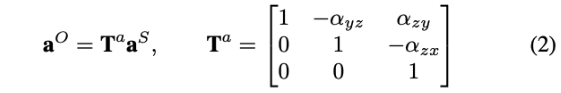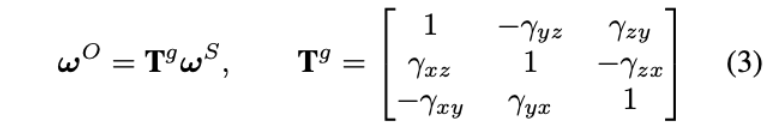• $$\omega^{\mathcal{O}}$$表示在AOF坐标系的角速度
• $$\omega^{\mathcal{S}}$$表示在GF坐标系的角速度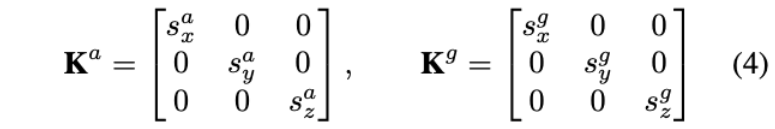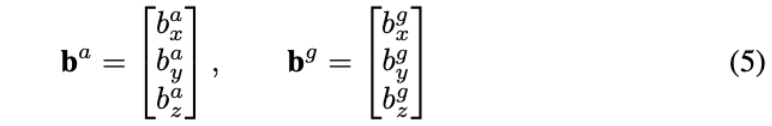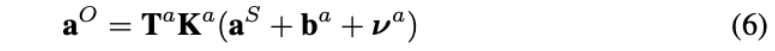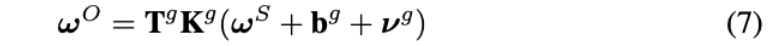• $$\nu^{a}$$表示加速度计测量噪声
• $$\nu^{g}$$表示陀螺仪测量噪声

# 标定Framework

## 加速度计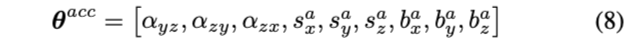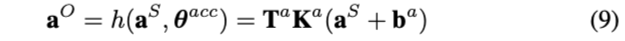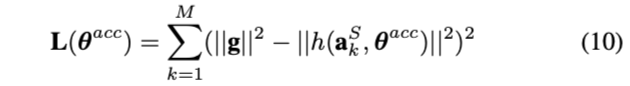• $$||g||$$表示当地重力向量的模（可以查表得到）

## 陀螺仪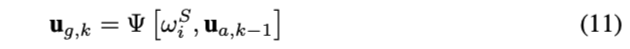$$\Psi$$可以使用任一种积分方法来得到k时刻的姿态。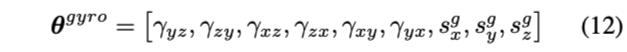cost function如下：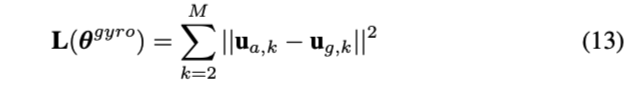• $$M$$表示由多少段是静态的
• $$u_{a,k}$$表示在第k段静态时间内，加速度计补偿输出值的平均值
• $$u_{g,k}$$表示使用等式(11)在[k-1,k]间隔内进行积分得到载体的姿态，然后把初始重力(即k-1时刻下的加速度计补偿输出值的平均值)进行投影得到的投影值。

# 标定过程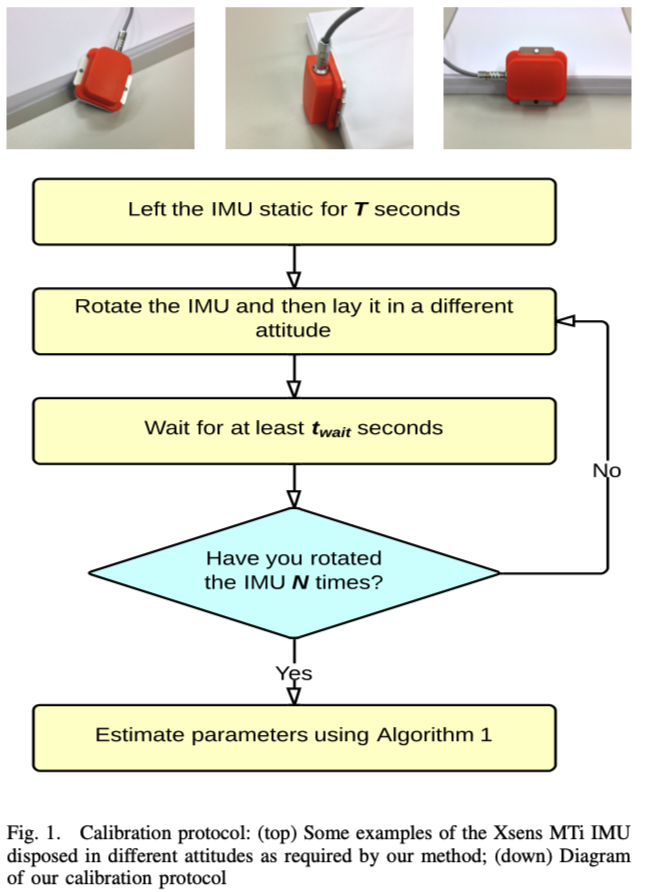• 为了校准加速度计，我们使用静态间隔（单段就可以）
• 而对于陀螺仪校准，除了两个连续的静态间隔，还包括两个连续的静态间隔之间的运动间隔(用来积分)

## 静态检测器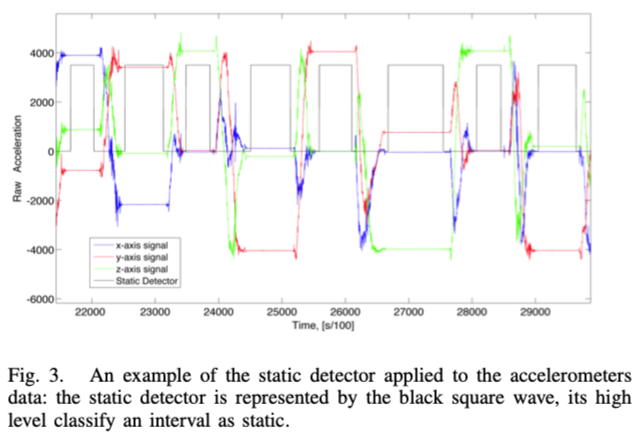。。。。具体见原文描述

## RK-4积分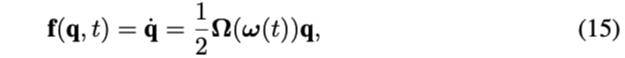• $$\Omega(\omega)$$表示把三轴角速度变成反对称矩阵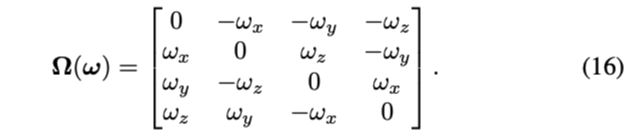rk4积分算法如下：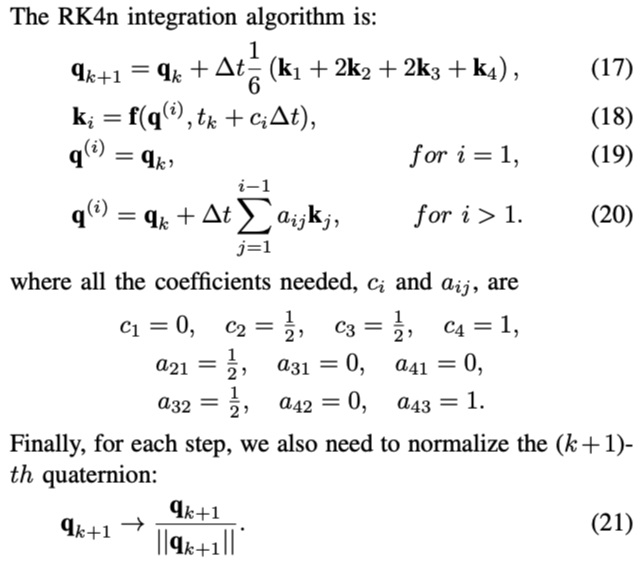## Allan方差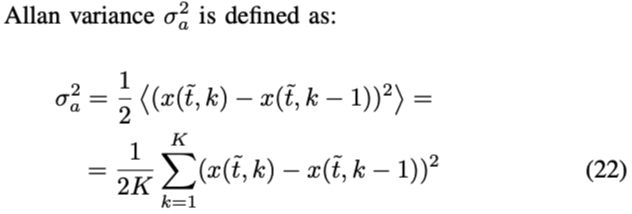• $$x(t,k)$$表示第k个静态间隔内平均值，跨度约为t秒
• K表示将考虑的总时间分割的间隔数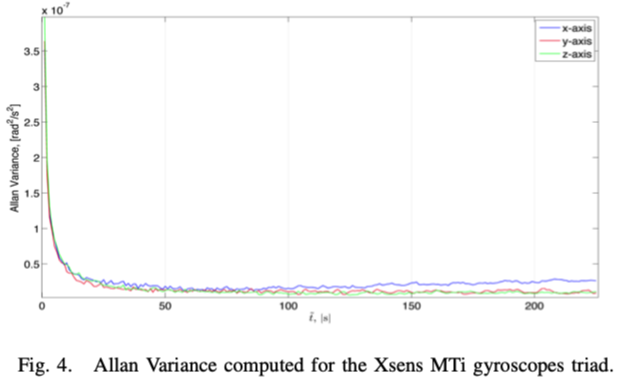## 完整过程

• 36<=N<=50
• 1s<=$$t_{wait}$$<=4s